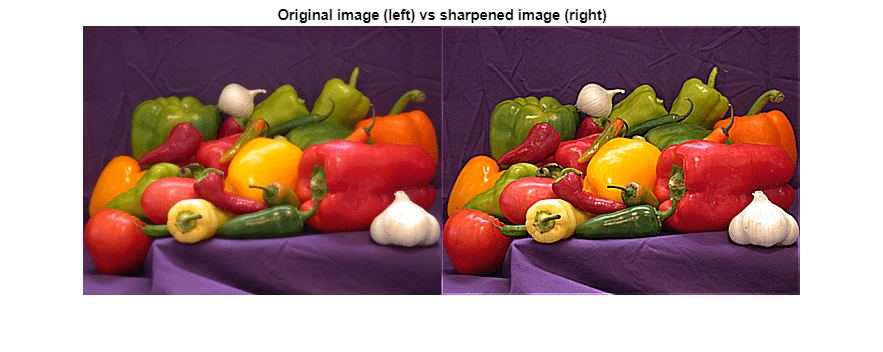# Sharpen an Image Using the GPU

This example shows how to sharpen an image using gpuArrays and GPU-enabled functions.

Read the image, and send it to the GPU using the `gpuArray` function.

`image = gpuArray(imread('peppers.png'));`

Convert the image to doubles, and apply convolutions to obtain the gradient image. Then, using the gradient image, sharpen the image by a factor of `amount`.

```dimage = im2double(image); gradient = convn(dimage,ones(3)./9,'same') - convn(dimage,ones(5)./25,'same'); amount = 5; sharpened = dimage + amount.*gradient;```

Resize, plot and compare the original and sharpened images.

```imshow(imresize([dimage, sharpened],0.7)); title('Original image (left) vs sharpened image (right)');```